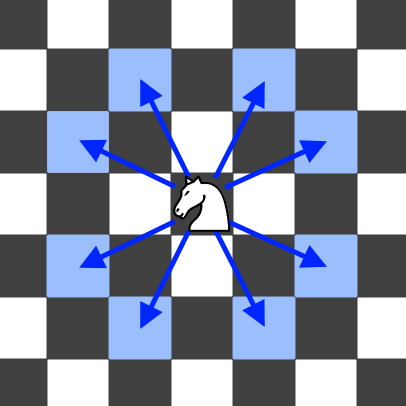| English | 简体中文 |

# 688. 骑士在棋盘上的概率

## 题目描述输入: n = 3, k = 2, row = 0, column = 0



输入: n = 1, k = 0, row = 0, column = 0



• 1 <= n <= 25
• 0 <= k <= 100
• 0 <= row, column <= n